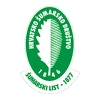DIGITALNA ARHIVA ŠUMARSKOG LISTA
prilagođeno pretraživanje po punom tekstu

 ŠUMARSKI LIST 7-8/2020 str. 61     <-- 61 -->        PDF Summary For assessment of growing stock in B&H forestry praxis as scientific baseline we are using volume tables and tariffs (Drinići dr. 1990). Having in mind the fact that these are constructed based on volume coefficients of the trees taken from German tree volume tables, which originate from single-age assortments, it is justified to suspect that by applying these tables one will get certain differences in quantity of large wood mass related to the actual status of those volumes. It is known that trees of the same tree species coming from single-age assortments are more full-bodied because they have larger volume coefficients compared to the trees of the same species from variable aged and selection forest assortments. That is why the objective of this research is to find “the best” regression model for levelling of volume of large wood of spruce, as dependent variable depending on diameter at breast height and tree height, as independent variables. To achieve this objective we have selected 377 model trees of spruce that were measured in felled condition on wider area of state owned variable aged assortments in Canton 10 (Livno Canton). To determine volume of large wood of trees we applied section method with sections of uneven absolute lengths (most often from 1 – 2 m). For levelling of volumes of large wood depending on diameter at breast height and tree height we applied method of multi-regression analysis. Quality of levelling and appropriateness of tested models was evaluated on the basis of determined values of basic statistical indicators for characterisation of the strength of correlation connections. The best values of the parameters showed model: V7=a0+a1d1,3+a2h+a3d1,3h+a4d1,32+a5 d1,32h with determined determination coefficient: R2 = 0,99 and size of standard regression error: Sey=0,245 m3. By testing significance of differences between actual/real tree volumes from the sample and volume of those trees determined by application of selected regression model by using t-test of pairs, no statistically significant differences were recorded. Average percentage of deviation was -0.44%. That means that in average we get 0.44% lower volumes comparing to the actual volumes in the sample of 377 spruce trees which shows that this regression model is usable for application in practice, because that average percentage is less than 1%. Statistically significant differences were recorded between volumes of trees of selected regression model and volume tables and models of other authors (Stojanović i dr. 1978; Banković i dr. 2003; Špiranec, 1976.). Those deviations vary in the range from 2.53% up to 22.7%. The largest deviations were determined exactly in model that is used in B&H (Stojanović i dr. 1978) and are in amount of 22.7% while the lower deviations were determined for volumes estimated by using model created for spruce trees from Sub-Alpine belt for area of Kopaonik (Banković i dr. 2003) and those amount to 2.53%. Key words: spruce, merchantable wood volume, regression model, nonlinear regression, two-entry volume tables